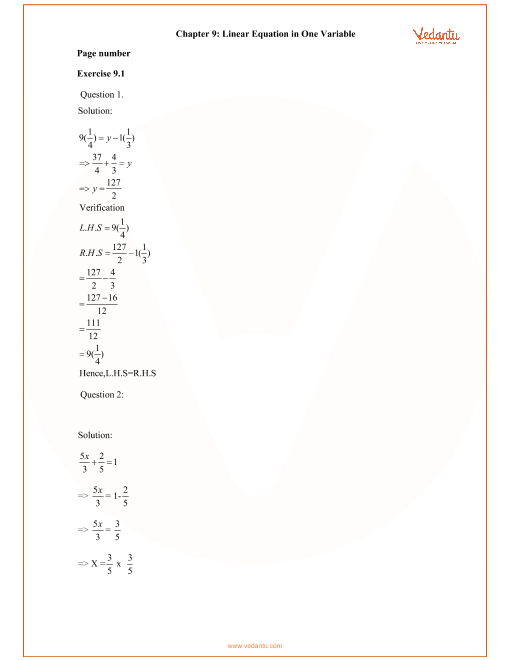RD Sharma Class 8 Solutions Chapter 9 - Linear Equation In One Variable (Ex 9.3) Exercise 9.3

RD Sharma Class 8 Solutions Chapter 9 - Linear Equation In One Variable (Ex 9.3) Exercise 9.3 - Free PDF

Free PDF download of RD Sharma Class 8 Solutions Chapter 9 - Linear Equation In One Variable Exercise 9.3 solved by Expert Mathematics Teachers on Vedantu.com. All Chapter 9 - Linear Equation In One Variable Ex 9.3 Questions with Solutions for RD Sharma Class 8 Maths to help you to revise complete Syllabus and Score More marks. Register for online coaching for IIT JEE (Mains & Advanced) and other Engineering entrance exams.